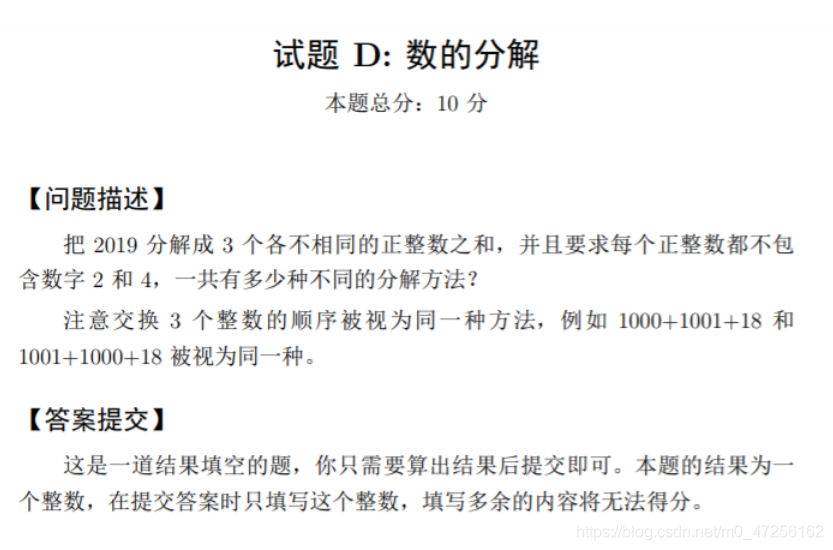[Inscription] Decomposition

[Problem Description]

Decompose 2019 into three different intensive sums, and require each positive integerDo not containNumbers 2 and 4, how many different decomposition methods?
Note that the order of exchange of three integers is considered as the same method, such as 1000 + 1001 + 18 and 1001 + 1000 + 18 is considered as the same.

[Submitted]

This is a question that fills the blanks. You only need to calculate the results will be submitted. The result of this question is an integer. Only the integers are filled in when the answer is submitted, and the extra content will not be score.Solution Idea

All arrangements, each case.

[Code]

public static void func () {INT n = 2019, count = 0; for (int i = 1; i <n; i ++) {// The first number skip contains 2 and 4 number IF ((i + "") .Contains ("2") || (i + "") .Contains ("4")) Continue; for (int J = i + 1; j <n; j ++) {// second Skip the number IF ((J + ") containing 2 and 4) .Contains (" 2 ") || (J +")) .Contains ("4"))) Continue; int temp = n - i - j; // Remove the number that does not meet the conditions. IF (Temp <= 0 || i == Temp || j == Temp) Continue; // The third number skips contains 2 and 4 number IF ((TEMP + ") .Contains (" 2 ") || (Temp + ") .Contains (" 4 "))) Continue; Count ++;}} system.out.printf (" A total of% D decomposition method. ", count / 3);}

Result

40785

• Top